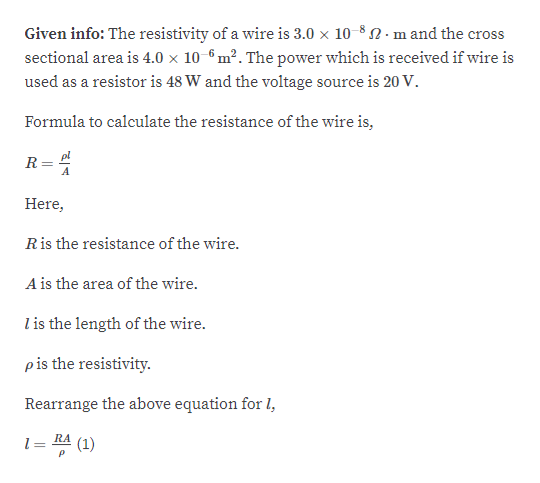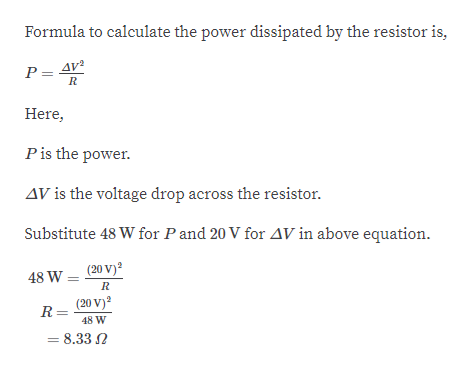# A particular wire has a resistivity of 3.0 x 10-8 Ω ⋅ m and across - sectional area of 4.0 x 10-6 m2. A length of this wire is tobe used as a resistor that will develop 48 W of power when connectedacross a 20. - V battery. What length of wire is required?

Question
9 views

A particular wire has a resistivity of 3.0 x 10-8 Ω ⋅ m and a
cross - sectional area of 4.0 x 10-6 m2. A length of this wire is to
be used as a resistor that will develop 48 W of power when connected
across a 20. - V battery. What length of wire is required?

check_circle

Step 1help_outlineImage TranscriptioncloseGiven info: The resistivity of a wire is 3.0 x 10-8N.m and the crss sectional area is 4.0 x 10-6 m². The power which is received if wire is used as a resistor is 48 W and the voltage source is 20 V. Formula to calculate the resistance of the wire is, pl R Here, Ris the resistance of the wire. A is the area of the wire. l is the length of the wire. pis the resistivity. Rearrange the above equation for l, l = RA (1) fullscreen
Step 2help_outlineImage TranscriptioncloseFormula to calculate the power dissipated by the resistor is, AV² Here, Pis the power. AV is the voltage drop across the resistor. Substitute 48 W for P and 20 V for AV in above equation. (20 V)? 48 W (20 V)? R = 48 W = 8.33 N fullscreen

### Want to see the full answer?

See Solution

#### Want to see this answer and more?

Solutions are written by subject experts who are available 24/7. Questions are typically answered within 1 hour.*

See Solution
*Response times may vary by subject and question.
Tagged in

### Current Electricity